GCSE Maths Algebra Inequalities

Linear Inequalities

# Linear Inequalities

Here we will learn about linear inequalities, including what linear inequalities are and how to solve them.

There are also linear inequalities worksheets based on Edexcel, AQA and OCR exam questions, along with further guidance on where to go next if you’re still stuck.

## What are linear inequalities?

Linear inequalities are inequalities where the power of the unknown in any algebraic expression is no higher than 1.

For example,

4x+1<13 which is read ‘4x+1 is less than 13’.

We can solve linear inequalities in the same way that we solve linear equations, by using inverse operations to isolate the variable.

The difference is that the answer will be a range of solutions rather than a single value.

The solution is x<3. This means that x is any value less than 3.

Notice that the inequality symbol remains the same throughout the working and for the answer.

The solution is x is less than 3. This means x could be any value less than 3 (for example, 2, \ 1.5, \ 1, \ 0, \ -1, \ -1000, etc.).

### What are linear inequalities?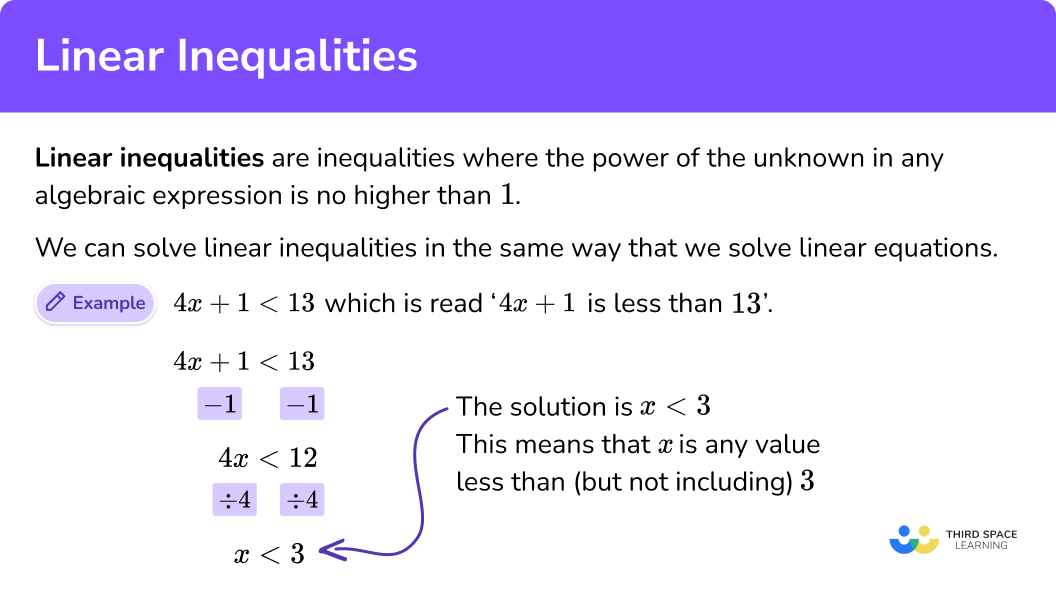### Negative numbers and inequalities

If you multiply or divide the inequality by a negative number, the direction of the inequality reverses.

For example, to solve the inequality -3x<30 we could divide both sides by -3.

Since multiplying or dividing by a negative reverses the direction of the inequality, this gives x>-10.

This only happens when we multiply or divide an inequality by a negative value.

## How to solve linear inequalities

In order to solve linear inequalities:

1. Rearrange the inequality so that all the unknowns are on one side of the inequality sign.
2. Rearrange the inequality by dividing by the \textbf{x} coefficient so that \textbf{‘x’} is isolated.
3. Write your solution with the inequality symbol.

### Explain how to solve linear inequalities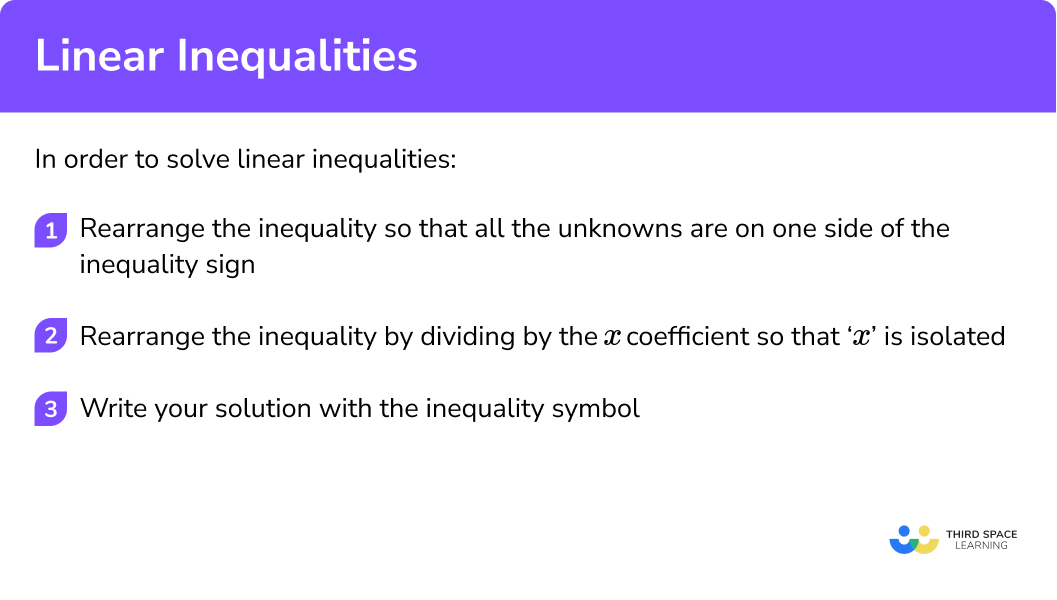## Related lessons on inequalities

Linear inequalities is part of our series of lessons to support revision on inequalities. You may find it helpful to start with the main inequalities lesson for a summary of what to expect, or use the step by step guides below for further detail on individual topics. Other lessons in this series include:

## Linear inequalities examples

### Example 1: one step linear inequality

Solve the inequality x-7>10.

1. Rearrange the inequality so that all the unknowns are on one side of the inequality sign.

In this case you are adding ‘7’ to both sides.

2Rearrange the inequality by dividing by the \textbf{x} coefficient so that \textbf{‘x’} is isolated.

In this case ‘x’ is already isolated.

3Write your solution with the inequality symbol.

In this example, we already have the solution, x>17.

### Example 2: two step linear inequality

Solve the inequality 5x+4\le 19.

Rearrange the inequality so that all the unknowns are on one side of the inequality sign.

Rearrange the inequality by dividing by the \textbf{x} coefficient so that \textbf{‘x’} is isolated.

Write your solution with the inequality symbol.

### Example 3: two step linear inequality with a fraction

Solve the inequality \frac{m}{3}+10<12.

Rearrange the inequality so that all the unknowns are on one side of the inequality sign.

Rearrange the inequality by dividing by the \textbf{x} coefficient so that \textbf{‘x’} is isolated.

Write your solution with the inequality symbol.

### Example 4: linear inequality with negative coefficient

Solve the inequality 20-3x<8.

Note: There are two ways to deal with negative inequalities. The first is to move the negative to the other side in order to make it positive. The second is to divide by the negative. Dividing by a negative reverses the direction of the inequality sign.

Let’s look at both ways of dealing with this.

Moving the negative to the other side

Step 1: Rearrange the inequality so that all the unknowns are on one side of the inequality sign.

Rearrange the inequality by dividing by the \textbf{x} coefficient so that \textbf{‘x’} is isolated.

Write your solution with the inequality symbol.

Dividing by a negative

Step 1: Rearrange the inequality so that all the unknowns are on one side of the inequality sign.

Rearrange the inequality by dividing by the \textbf{x} coefficient so that \textbf{‘x’} is isolated.

Write your solution with the inequality symbol.

Both methods give the same solution, x>4.

### Example 5: compound inequality

Solve the inequality -3<4x+1\le 17.

Rearrange the inequality so that all the unknowns are on one side of the inequality sign.

Rearrange the inequality by dividing by the \textbf{x} coefficient so that \textbf{‘x’} is isolated.

Write your solution with the inequality symbol.

### Example 6: linear inequality with brackets

Solve the inequality 6(y-3)\le 42.

Rearrange the inequality so that all the unknowns are on one side of the inequality sign.

Rearrange the inequality by dividing by the \textbf{x} coefficient so that \textbf{‘x’} is isolated.

Write your solution with the inequality symbol.

### Example 7: linear inequality with unknowns on both sides

Solve the inequality 5t+7>9t-13.

Rearrange the inequality so that all the unknowns are on one side of the inequality sign.

Rearrange the inequality by dividing by the \textbf{x} coefficient so that \textbf{‘x’} is isolated.

Write your solution with the inequality symbol.

### Example 8: linear inequality with brackets and unknowns on both sides

Solve the inequality 2(p+4)<6(4-p).

Rearrange the inequality so that all the unknowns are on one side of the inequality sign.

Rearrange the inequality by dividing by the \textbf{x} coefficient so that \textbf{‘x’} is isolated.

Write your solution with the inequality symbol.

### Common misconceptions

• Dropping the inequality

When solving inequalities students may use the same method as solving equations. During this process the inequality sign may be mixed up with the equality sign (=). It is important to ensure that the correct sign is used.

• Using the wrong operation

When working with equations and inequalities you need to remember to use the inverse operation.

For example, to solve x+8<14, you need to subtract 8 from both sides not add 8 to both sides.

• Performing the operation to the wrong side

Make sure you perform the operation to the correct side of an inequality.

For example, to solve \frac{x}{3}\ge12, multiplying by 3 gives x\ge 36, not 3x\ge 12.

### Practice linear inequalities questions

1. Solve the inequality 5x<30.

x=6x<6x<25x=150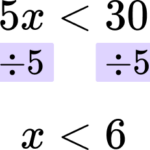2. Solve the inequality 8x-5>27.

x<4x>4x<2.75x>2.75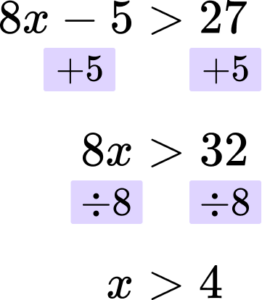3. Solve the inequality \frac{x}{6}+2\ge 5.

x=18x \ge 0.5x \ge 18x=42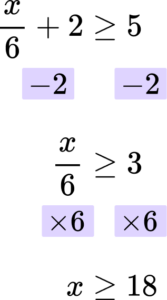4. Solve the inequality 30-3x\le 27.

x \le 9x \ge 9x \le 1x \ge 1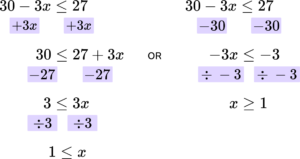5. Solve the inequality 13<5x-2\le 38.

3 \le x<83<x \le 8x \le 55 \le x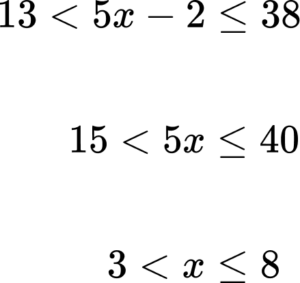6. Solve the inequality 2(3x-1)<46.

x<288x>288x<8x \le 8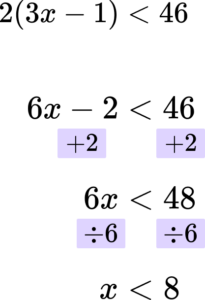7. Solve the inequality 8x-2>5x+4.

x>2x=2x>6x<6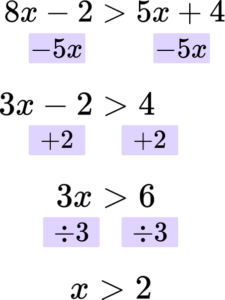8. Solve the inequality 2(3x-2)\le 14(4-x).

x \le 7.5x=7.5x=3x \le 3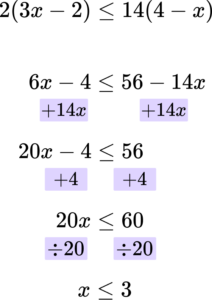### Linear inequalities GCSE questions

1. Solve the following inequality 3(4y+1)<63.

(2 marks)

12y+3<63

(1)

12y<60

y<5

(1)

2. (a) Solve the inequality 2x+5\ge3.

(b) Represent the solution set on the number line below.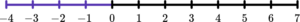(3 marks)

(a)

2x \ge -2

(1)

x \ge -1

(1)

(b)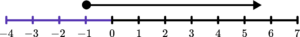Correct diagram with closed circle.

(1)

3. n is an integer such that 5<2n+1\le 13.

Write down all the possible values of n.

(3 marks)

4<2n\le12

(1)

2<n\le6

(1)

n could be 3, \ 4, \ 5, \ 6.

(1)

## Learning checklist

You have now learned how to:

• Solve linear inequalities including ones with brackets and unknowns on both sides
• Solve three-part linear inequalities

## Still stuck?

Prepare your KS4 students for maths GCSEs success with Third Space Learning. Weekly online one to one GCSE maths revision lessons delivered by expert maths tutors.

Find out more about our GCSE maths tuition programme.